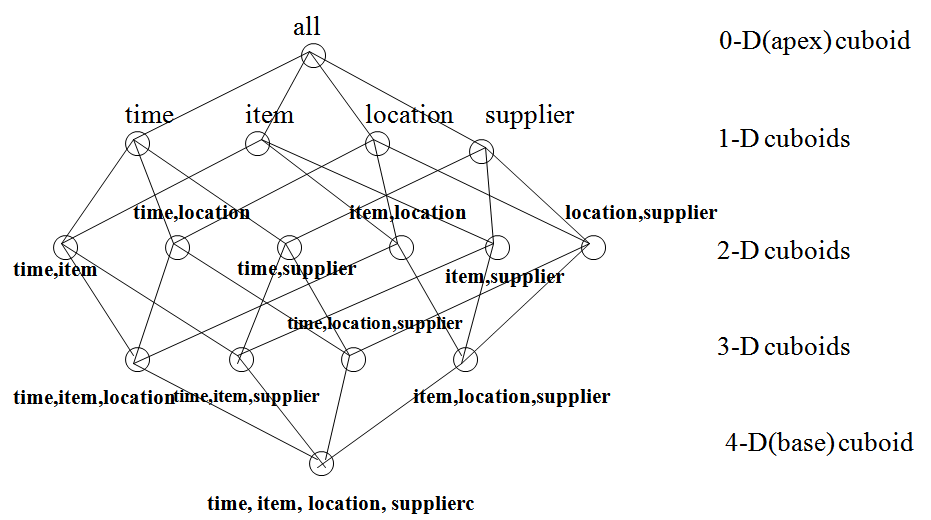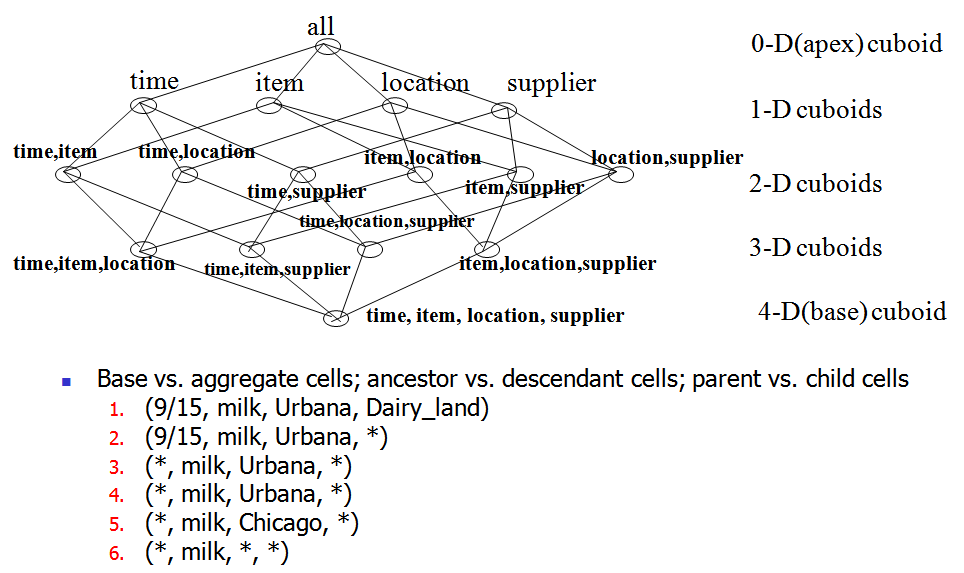### Data Cube: A Lattice of Cuboids### Data Cube: A Lattice of Cuboids### Cube Materialization: Full Cube vs. Iceberg Cube• Full cube vs. iceberg cube
• compute cube sales iceberg as
• select month, city, customer group, count(*)
• from salesInfo
• cube by month, city, customer group
• iceberg condition: having count(*) >= min support
• Computing only the cuboid cells whose measure satisfies the iceberg condition
• Only a small portion of cells may be “above the water’’ in a sparse cube
• Avoid explosive growth: A cube with 100 dimensions
• 2 base cells: (a1, a2, …., a100), (b1, b2, …, b100)
• How many aggregate cells if “having count >= 1”?
• What about “having count >= 2”?

### Iceberg Cube, Closed Cube & Cube Shell

• Is iceberg cube good enough?
• 2 base cells: {(a1, a2, a3 . . . , a100):10, (a1, a2, b3, . . . , b100):10}
• How many cells will the iceberg cube have if having count(*) >= 10? Hint: A huge but tricky number!
• Close cube:
• Closed cell c: if there exists no cell d, s.t. d is a descendant of c, and d has the same measure value as c.
• Closed cube: a cube consisting of only closed cells
• What is the closed cube of the above base cuboid? Hint: only 3 cells
• Cube Shell
• Precompute only the cuboids involving a small # of dimensions, e.g., 3
• More dimension combinations will need to be computed on the fly
• For (A1, A2, … A10), how many combinations to compute?

### Roadmap for Efficient Computation

• General cube computation heuristics (Agarwal et al.’96)
• Computing full/iceberg cubes: 3 methodologies
• Bottom-Up: Multi-Way array aggregation (Zhao, Deshpande & Naughton, SIGMOD’97)
• Top-down:
• BUC (Beyer & Ramarkrishnan, SIGMOD’99)
• H-cubing technique (Han, Pei, Dong & Wang: SIGMOD’01)
• Integrating Top-Down and Bottom-Up:
• Star-cubing algorithm (Xin, Han, Li & Wah: VLDB’03)
• High-dimensional OLAP: A Minimal Cubing Approach (Li, et al. VLDB’04)
• Computing alternative kinds of cubes:
• Partial cube, closed cube, approximate cube, etc.

### General Heuristics (Agarwal et al. VLDB’96)

• Sorting, hashing, and grouping operations are applied to the dimension attributes in order to reorder and cluster related tuples
• Aggregates may be computed from previously computed aggregates, rather than from the base fact table
• Smallest-child: computing a cuboid from the smallest, previously computed cuboid
• Cache-results: caching results of a cuboid from which other cuboids are computed to reduce disk I/Os
• Amortize-scans: computing as many as possible cuboids at the same time to amortize disk reads
• Share-sorts: sharing sorting costs cross multiple cuboids when sort-based method is used
• Share-partitions: sharing the partitioning cost across multiple cuboids when hash-based algorithms are used

Creator: sidraaslam

Contributors:
-

Licensed under the Creative Commons
Attribution ShareAlike CC-BY-SA license

This deck was created using SlideWiki.